Courses
Courses for Kids
Free study material
Free LIVE classes
More

# Purely Resistive, Purely Inductive and Purely Capacitive Circuits for JEELIVE
Join Vedantu’s FREE Mastercalss

## Significance of Purely Resistive, Purely Inductive and Purely Capacitive Circuits

An AC circuit consists of a combination of capacitance C, inductance L, and resistance R. In order to better understand the effect of each of the above parameters in a general AC circuit, it is important to consider these parameters in isolation. Hence, pure resistive, pure inductive, and pure capacitive circuits are more like an experiment rather than an actual practice. In this section, let’s look at the characteristics and phasor diagrams of pure resistive, pure inductive, and pure capacitive circuits.

## Purely Resistive Circuit

Definition: A purely resistive circuit has a very negligible amount of inductance such that the reactance offered by such circuits is very small when compared to the resistance even at normal frequency.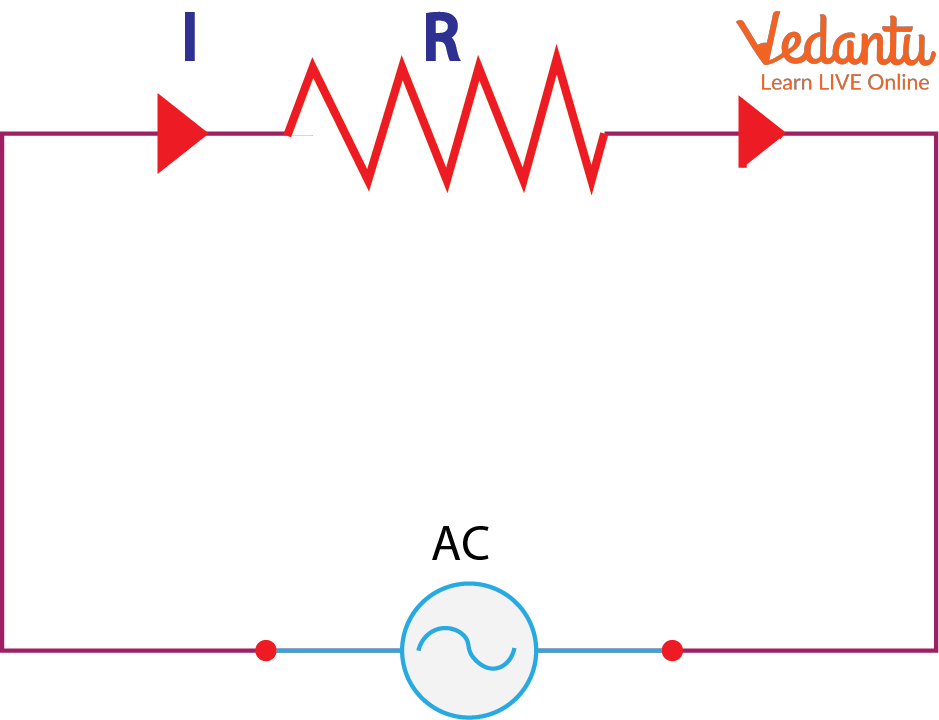Purely Resistive Circuit Diagram

The entire voltage applied over this circuit is exploited in overcoming the extremely high amount of resistance. Hence, these are often called non-inductive circuits.

Properties

• No presence of inductance and capacitance.

• The backward movement of both the alternating current and the voltage can be observed. Hence, they form a sine wave or sinusoidal waveform.

• The value of current and voltage reaches the maximum at the same time.

• The resistor is a passive device in such a circuit that converts the electrical energy into heat.

Phasor Diagram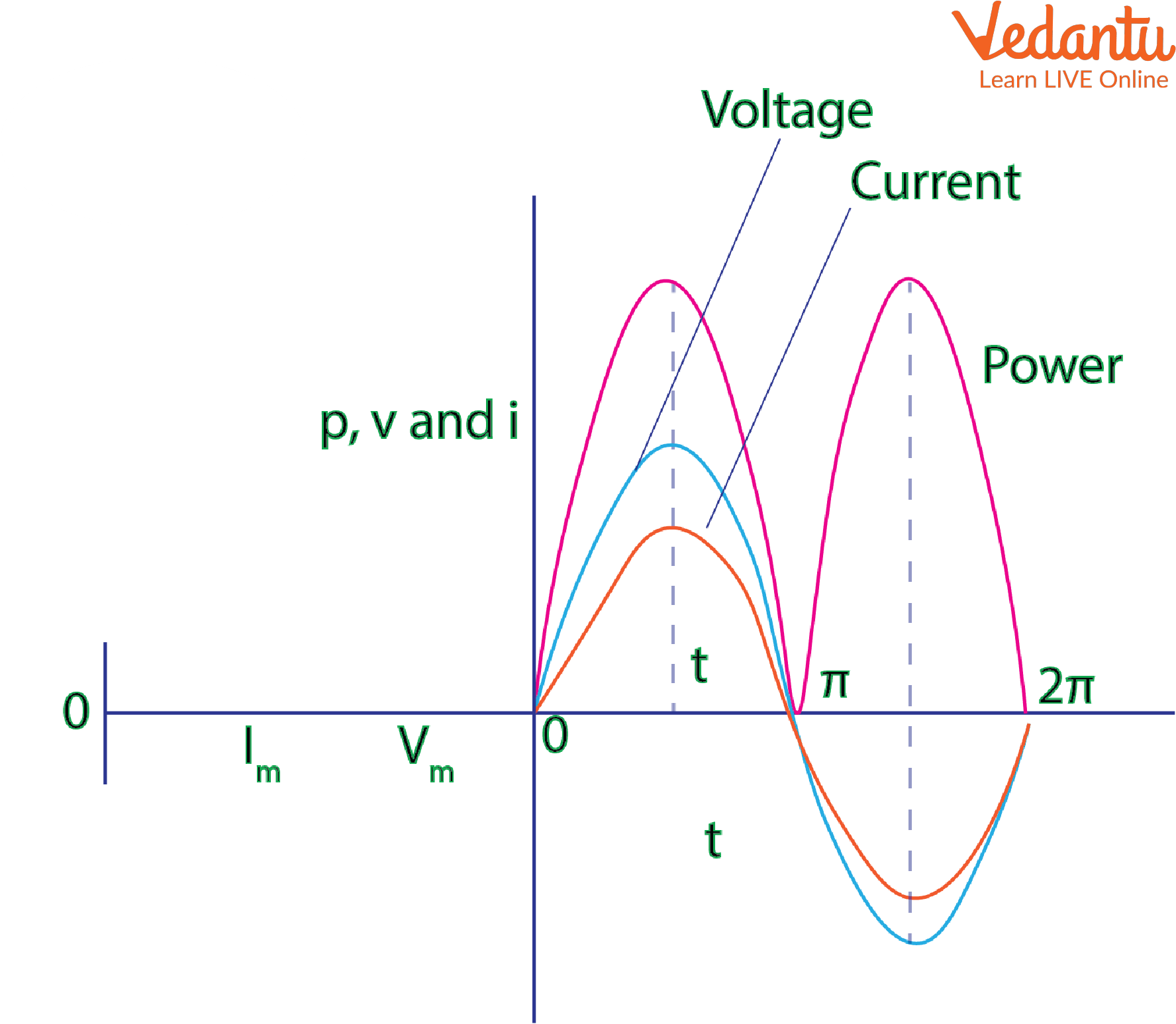Purely Resistive Circuit Phasor and Waveform Diagram

Derivation of Formulas

The equation of the alternating voltage that is applied across the circuit is

$v={{V}_{m}}\sin \omega t$  …….. (1)

The current flowing through the purely resistive circuit can be derived as

$i=\frac{V}{R}=\frac{{{V}_{m}}}{R}\sin \omega t$   ………. (2)

$i={{I}_{m}}\sin \omega t$         …….. (3)

From (1) and (3), it is clear that no phase difference exists between the voltage applied and the current flowing through a purely resistive circuit. Hence, the current is in phase with the voltage and the phase angle is zero. As a result, both the current and the voltage attain a peak at the same time and for all values of current and voltage, the power curve (shown in the above graph in pink, blue, and red) is positive.

Hence, the equation for instantaneous power is p = vi

$p=\left( {{V}_{m}}\sin \omega t \right)\left( {{I}_{m}}\sin \omega t \right)$

$p=\frac{\left( {{V}_{m}}{{I}_{m}} \right)}{2}2{{\sin }^{2}}\omega t=\frac{{{V}_{m}}}{\sqrt{2}}\frac{{{I}_{m}}}{\sqrt{2}}\left( 1-\cos 2\omega t \right)$

The average power consumption over a complete cycle in a purely resistive circuit is given by

$p=avg\left( \frac{{{V}_{m}}}{\sqrt{2}}\frac{{{I}_{m}}}{\sqrt{2}} \right)-avg\left( \frac{{{V}_{m}}}{\sqrt{2}}\frac{{{I}_{m}}}{\sqrt{2}}\cos 2\omega t \right)$

The maximum current will be when $\omega t={{90}^{\circ }}$ and hence $\cos {{90}^{\circ }}=0$

$P={{V}_{rms}}{{I}_{rms}}$

where ${{V}_{rms}}$  and ${{I}_{rms}}$ are root mean square values of supply voltage and current, respectively, and P is the average power.

Hence, the power in a purely resistive circuit is given by P = VI

## Purely Inductive Circuit

Definition

Such a circuit theoretically has zero resistance and hence zero loss. A back EMF due to the self-inductance of the coil is produced whenever alternating voltage is applied to a purely inductive circuit. Due to the absence of ohmic resistance, the only force that the applied voltage has to overcome is the circuit’s self-inductance.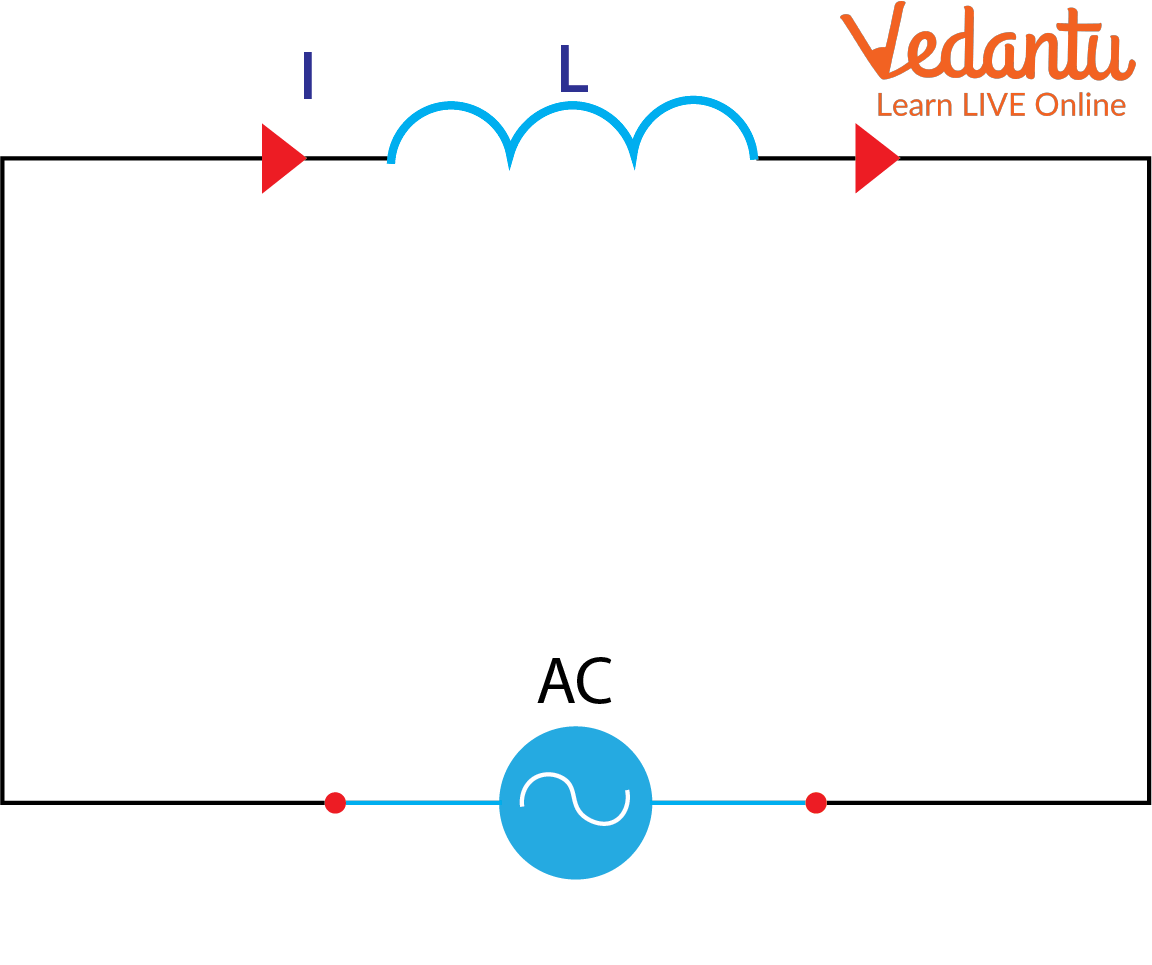Purely Inductive Circuit Diagram

Properties

• When the voltage and current values are at their positive peak, the value of power is also positive and similarly, when the voltage and current values are at their negative peak, power is also negative.

• The current value changes during a voltage drop and at the instant when the current value is at its peak, the voltage will reach zero.

• The voltage and current are out of phase with each other by 90o.

Phasor and Wavelength Diagram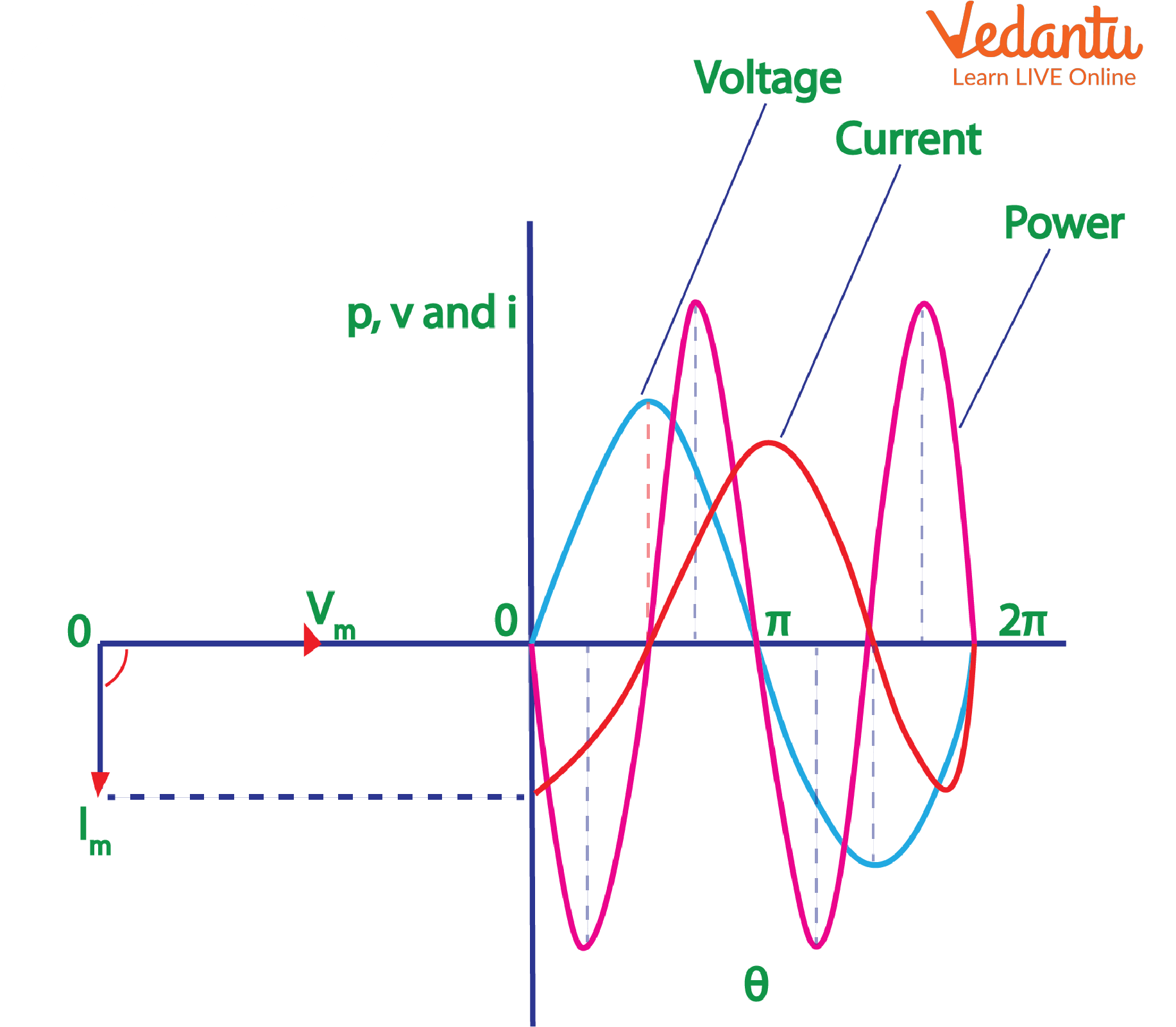Purely Inductive Circuit Phasor and Waveform Diagram

Derivation of Formulas

The equation of the alternating voltage that is applied across the circuit is

$v={{V}_{m}}\sin \omega t$                 ………. (1)

This results in  current flow along with an induced emf given by

$e=-L\frac{di}{dt}$

Since the induced emf is equal and opposite to the applied voltage,

$v=-\left( -L\frac{di}{dt} \right)$

${{V}_{m}}\sin \omega t=L\frac{di}{dt}$

$di=\frac{{{V}_{m}}}{L}\sin \omega tdt$           ……… (2)

Integrating both sides of (2) we get,

$i=\frac{{{V}_{m}}}{\omega L}\sin \left( \omega t-\frac{\pi }{2} \right)=\frac{{{V}_{m}}}{{{X}_{L}}}\sin \left( \omega t-\frac{\pi }{2} \right)$                         ………. (3)

where XL is the opposition offered by pure inductance to the flow of alternating current and is called inductive reactance.

The maximum current will be when $\sin \left( \omega t-\frac{\pi }{2} \right)=1$. Hence,

${{I}_{m}}=\frac{{{V}_{m}}}{{{X}_{L}}}$        ……… (4)

Substituting (3) in (4) we get,

$i={{I}_{m}}\sin \left( \omega t-\frac{\pi }{2} \right)$

The instantaneous power in a purely inductive circuit can be derived as follows:

$P = VI$

$P=\left( {{V}_{m}}\sin \omega t \right){{I}_{m}}\sin \left( \omega t+\frac{\pi }{2} \right)$

$P={{V}_{m}}{{I}_{m}}\sin \omega t\cos \omega t$

$P=\frac{{{V}_{m}}{{I}_{m}}}{2}2\sin \omega t\cos \omega t$

$P=\frac{{{V}_{m}}}{\sqrt{2}}\frac{{{I}_{m}}}{\sqrt{2}}\sin 2\omega t$

Or P = 0 i.e., average power consumption in a purely inductive circuit is zero.

## Purely Capacitive Circuit - Definition, Phasor Diagram, and Derivation of Formula

Definition: In this type of circuit, upon supply of alternative voltage, the capacitor gets charged first in one direction and next in another direction. Hence, it charges itself when there is supply and discharges when there is no supply.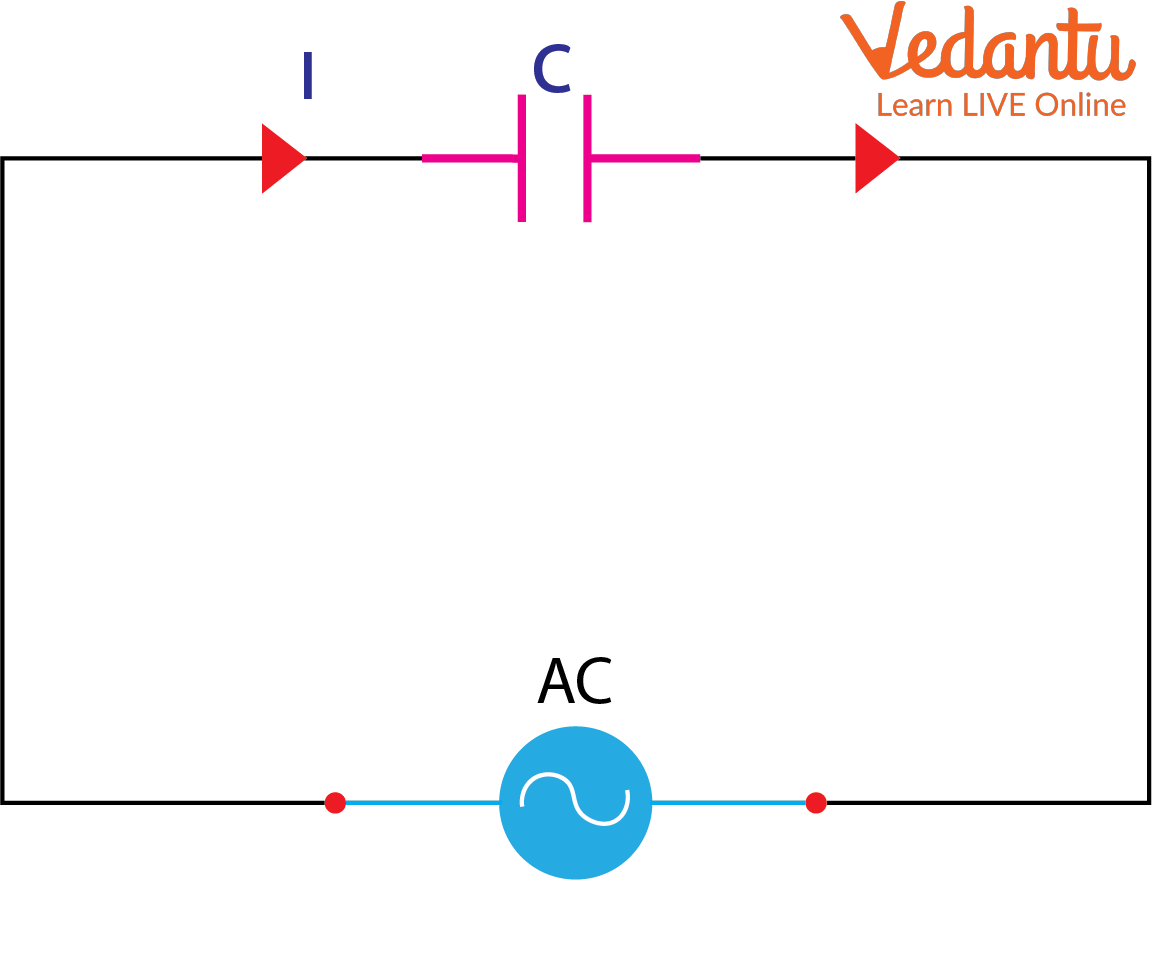Purely Capacitive  Circuit Diagram

Properties

• Current leads voltage by a phase difference of 90o.

• When there is an increase in voltage, the positive half cycle begins, i.e., the capacitor gets charged and reaches its maximum capacity.

• Similarly, when there is a decrease in voltage, the negative half cycle happens leading to discharge from the capacitor.

• During maximum voltage, current flow is zero.

• Current starts to flow when voltage value decreases to a negative value.

Phasor and Wavelength Diagram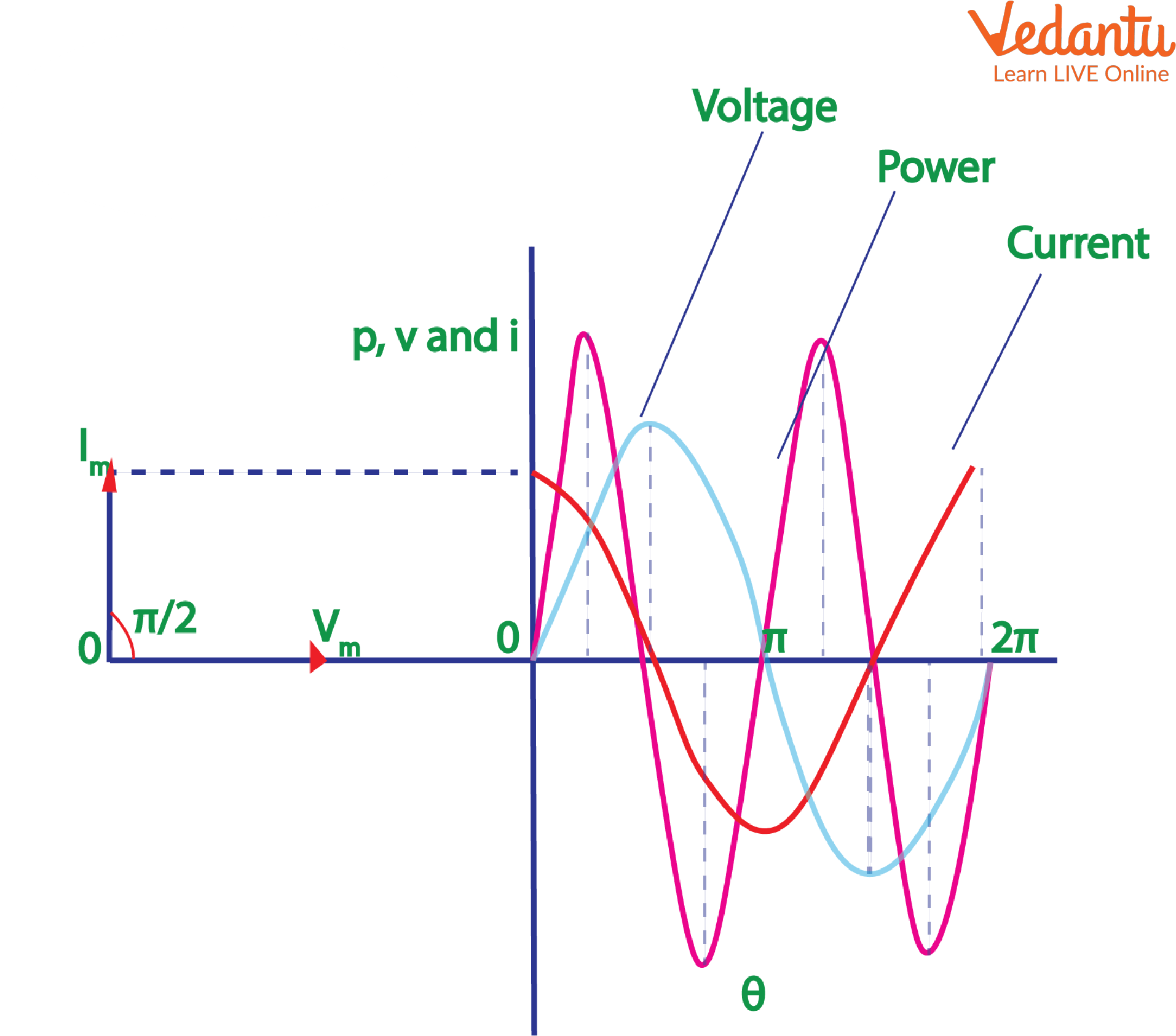Purely Capacitive Circuit Phasor and Waveform Diagram

Derivation of Formulas

The equation of the alternating voltage that is applied across the circuit is

$v={{V}_{m}}\sin \omega t$    …. (1)

At any point in time, let the charge of the capacitor be

$q=Cv$        …. (2)

The current flowing through the purely capacitive circuit is given by:

$i=\frac{dq}{dt}$

Substituting (2) in the above equation,

$i=\frac{d}{dt}\left( Cv \right)$ …. (3)

$i=\frac{d}{dt}C{{v}_{m}}\sin \omega t=C{{v}_{m}}\frac{d}{dt}\sin \omega t$

$i=\omega C{{v}_{m}}\cos \omega t=\frac{{{V}_{m}}}{1/\omega C}\sin \left( \omega t+\frac{\pi }{2} \right)$

$i=\frac{{{V}_{m}}}{{{X}_{C}}}\sin \left[ \omega t+\frac{\pi }{2} \right]$ …. (4)

where is ${{X}_{C}}=1/\omega C$ the capacitive reactance

Current will be maximum when  $\sin \left( \omega t+\frac{\pi }{2} \right)=1$. Hence,

${{I}_{m}}=\frac{{{V}_{m}}}{{{X}_{C}}}$

On substituting this in (4) we get,

$i={{I}_{m}}\sin \left( \omega t+\frac{\pi }{2} \right)$

The instantaneous power in the capacitor P = VI

$P=\left( {{V}_{m}}\sin \omega t \right){{I}_{m}}\sin \left( \omega t+\frac{\pi }{2} \right)$

$P={{V}_{m}}{{I}_{m}}\sin \omega t\cos \omega t$

$P=\frac{{{V}_{m}}}{\sqrt{2}}\frac{{{I}_{m}}}{\sqrt{2}}\sin 2\omega t$

Or P = 0, i.e., average power consumption in a purely inductive circuit is zero.

## Summary

In order to understand a general AC circuit, the capacitance, inductance, and resistance factors must be studied in isolation. It will yield important results about the relationship between current and voltage, the phase difference, and the average power consumption.

Last updated date: 26th Sep 2023
Total views: 2.1k
Views today: 0.02k

## FAQs on Purely Resistive, Purely Inductive and Purely Capacitive Circuits for JEE

1. What are the steps in finding the impedance in a purely resistive circuit connected to another current element?

Individual resistances of all the elements are calculated. We know that in a purely resistive circuit, current and voltage are in phase with each other, and in an inductor, voltage leads the current. As the final step, the net impedance of the circuit is found. The phase angle of impedance is zero degrees and minus ninety degrees in a purely resistive and a purely capacitive circuit, respectively. However, in the case of an AC Capacitance where resistance and capacitance are present in the same circuit, the phase angle will be between the values mentioned for pure circuits.

2. Briefly comment on the relationship between capacitive reactance and frequency.

The amount of charging that can be done in a capacitor is called its capacitive reactance. In other words, capacitive reactance can be taken as the opposition offered to the flow of alternating current by the capacitor. With an increase in the frequency between its two plates, the capacitive reactance will decrease and hence it establishes an inversely proportional relationship with the frequency.  The AC capacitance value of a capacitor remains constant despite the opposition offered to current flow by the capacitive reactance.